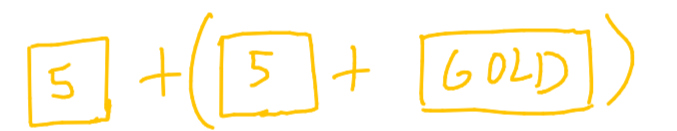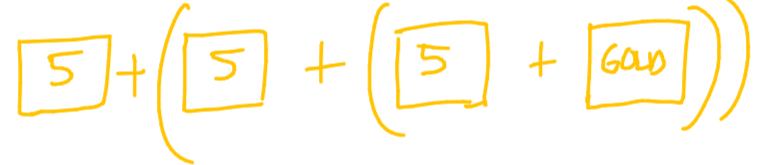# Chapter 2: Splitting Bars

In step, we saw how we could use recursive functions to methodically shrink the problem until it was gone. This approach is useful for basic problems like step, but we're going to get more advanced...

### Recursive problem #2: Split

Imagine a bar of solid gold.You want to find out how much it weighs, but you're held back by one thing:
You only know that a square of gold weighs 5 pounds.
Your gold bar is larger than a square.
As we did before, let's go ahead and define a function. The function split has one parameter: gold_bar, the bar of gold.
split aims to return how many pounds the gold bar weighs.
def split(gold_bar):
#this function should return how much "gold_bar" weighs!
If our problem is weighing the gold bar, let's think about how we can make the problem smaller! True to the function's name, why don't we try splitting the gold bar...
In this case, we can split the gold bar into:square_of_gold + (rest_of_bar)
The square of gold is a known quantity: we know it weighs 5 pounds. The rest of the bar is an unsolved problem. However, it's smaller than our original problem! It's important to realize that when added together, these two smaller problems are equivalent to our original problem, but they're easier for us to solve.
We can take the rest of the bar and split it again, and repeat this process. What does this look like in code?
def split(gold_bar):
return 5 + split(rest_of_bar)
We keep splitting the rest of the bar in order to make our problem smaller.
split(gold_bar) -> split(rest_of_bar) -> split(rest of rest_of_bar) -> ...
Again, because we are making the problem smaller and smaller; this line is the recursive call.
For now, let's continue splitting.square_of_gold + split(rest_of_bar)
square_of_gold + (square_of_gold + split(rest_of_bar))
And split the rest of the bar again....We are left with
square_of_gold + ( square_of_gold + (square_of_gold + split(rest_of_bar))
but the rest of the bar is a square! That's a known quantity! This is equivalent tosquare_of_gold + ( square_of_gold + (square_of_gold + square_of_gold))
Which is 5 + 5 + 5 + 5 = 20 pounds!
By now you should have realized that our base case is when we have split the gold bar enough times so that it is a square, a known quantity. In code:
def split(gold_bar):
if gold_bar == square_of_gold:
return 5
return 5 + split(rest_of_bar)
Now, every time we split, we see if the gold_bar we're splitting is a square; if it is, we return it, and we're finished! Otherwise, we return square of gold + split(rest of bar), knowing that split will make our problem smaller.
If you're still confused or not completely sold, here's a quick recap to show you what's happening:
split(gold_bar) -> square + split(rest_of_bar) #4 squares = 20 pounds
V
(square + split(rest_of_previous_bar)) #3 squares
V
(square + split(rest_of_previous_bar)) #2 squares
V
square

### Great, now what?

So, what was the point of this example? The goal of this example was to introduce the idea of splitting a problem into two smaller ones; In our example, we split the problem of weighing a gold bar into the problems of:
1. 1.
Weighing a square of gold
2. 2.
Weighing the rest of the bar
The first problem we could solve easily, and the second could be split further. In order to generalize this kind of thinking, consider this splitting strategy as splitting a problem into:
1. 1.
A solved or easily solvable problem
2. 2.
A problem that can be further split
I'll give concrete examples in the next chapter, to show you how we can use this approach in real code.